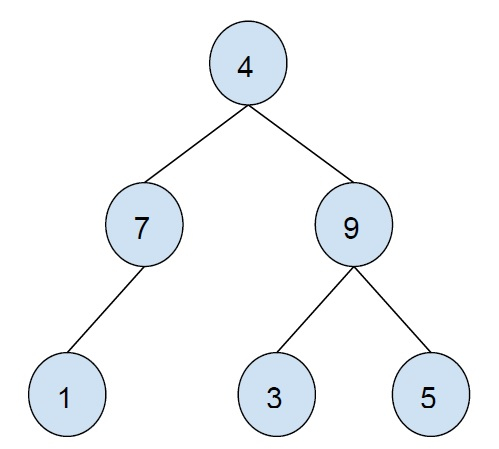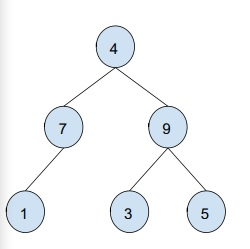# Print all full nodes in a Binary Tree in C++

In this problem, we are given a binary tree. Our task is to print all nodes of the tree that are full nodes.

The binary tree is a tree in which a node can have a maximum of 2 child nodes. Node or vertex can have no nodes, one child or two child nodes.

ExampleA full node is a node that has both its left and right child available. In other words, a node with the left and right child is a full node. In the above binary tree, 4 and 9 are full nodes.

Let’s take an example to understand the problem −Output − 4 9

A simple and easy approach to solve this problem is to traverse the tree using any traversal algorithm. Check if the current node has left and right children or node. If yes then print the node’s value otherwise leave it.

## Example

Program to illustrate our solution,

Live Demo

#include <iostream>
using namespace std;
struct Node{
int data;
struct Node *left, *right;
};
Node *insertNode(int data){
Node *temp = new Node;
temp->data = data;
temp->left = temp->right = NULL;
return temp;
}
void printFullNode(Node *root){
if (root != NULL){
printFullNode(root->left);
if (root->left != NULL && root->right != NULL)
cout<<root->data<<"\t";
printFullNode(root->right);
}
}
int main(){
Node* root = insertNode(100);
root->left = insertNode(56);
root->right = insertNode(12);
root->left->left = insertNode(89);
root->right->left = insertNode(32);
root->right->right = insertNode(45);
cout<<"All full nodes of the tree are :\n";
printFullNode(root);
return 0;
}

## Output

All full nodes of the tree are −
100 12